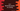# C++ program to find out the total vowels in a string# Introduction :

In this C++ programming tutorial, we will learn how to find out the total number of vowels in a string. The program will take one string as input from the user and it will print out the vowels.

With this program, you will learn how to read user inputs, how to print values to the user and how to use switch case in C++.

## Algorithm :

The algorithm of the program works like below :

1. Ask the user to enter a string.

2. Read and store the string in a variable.

3. Iterate through the characters of the string one by one.

4. Initialize one variable as 0 to store the total count of vowels.

5. For each character of the string, check if it vowel or not.

6. If it is a vowel, increment the count variable by 1.

7. Finally, print out the count variable to the user.

That’s it. Now, let’s write the above steps in code :

## C++ program :

``````#include <iostream>
using namespace std;

int main()
{
//1
char str;
int count = 0;

//2
cout << "Enter a string to test :" << endl;
cin.get(str, 100);

//3
for (int i = 0; str[i] != '\0'; i++)
{
//4
switch (str[i])
{
case 'a':
case 'e':
case 'i':
case 'o':
case 'u':
case 'A':
case 'E':
case 'I':
case 'O':
case 'U':
count++;
}
}

//5
cout << "Total number of vowels found :  " << count << endl;
}
``````

### Explanation :

The commented numbers in the above program denote the step numbers below :

1. Create one character array str to hold the user input string. Also, create one integer variable count to hold the total count of vowels.

2. Ask the user to enter the string. Read the user input string using cin and store it in the str variable.

3. Iterate through the characters of the string one by one using a for loop. This loop will run till the end of the string is reached or it finds the ‘\0’ character in the array.

4. Run one switch case to check if the current character is vowel or not. This switch block has 10 different case statements to check if the current character is a vowel or not. If a vowel is found, increment the value of count by 1. If it is not a vowel, move to the next iteration of the loop.

5. Finally, after the loop is completed, print the number of vowels found, i.e. the value of the variable count.

### Sample Output :

``````Enter a string to test :
AeIoUaEiOuhelloworld
Total number of vowels found : 13

Enter a string to test :
hello
Total number of vowels found : 2
``````

## Using an if-else condition :

We can also solve this problem using an if-else condition like below :

``````#include <iostream>
using namespace std;

int main()
{
char str;
int count = 0;

cout << "Enter a string to test :" << endl;
cin.get(str, 100);

for (int i = 0; str[i] != '\0'; i++)
{
if (str[i] == 'a' || str[i] == 'e' || str[i] == 'i' || str[i] == 'o' || str[i] == 'u' ||
str[i] == 'A' || str[i] == 'E' || str[i] == 'I' || str[i] == 'O' || str[i] == 'U')
{
count++;
}
}

cout << "Total number of vowels found :  " << count << endl;
}
``````

The output is the same as the above example.